九路85 0 0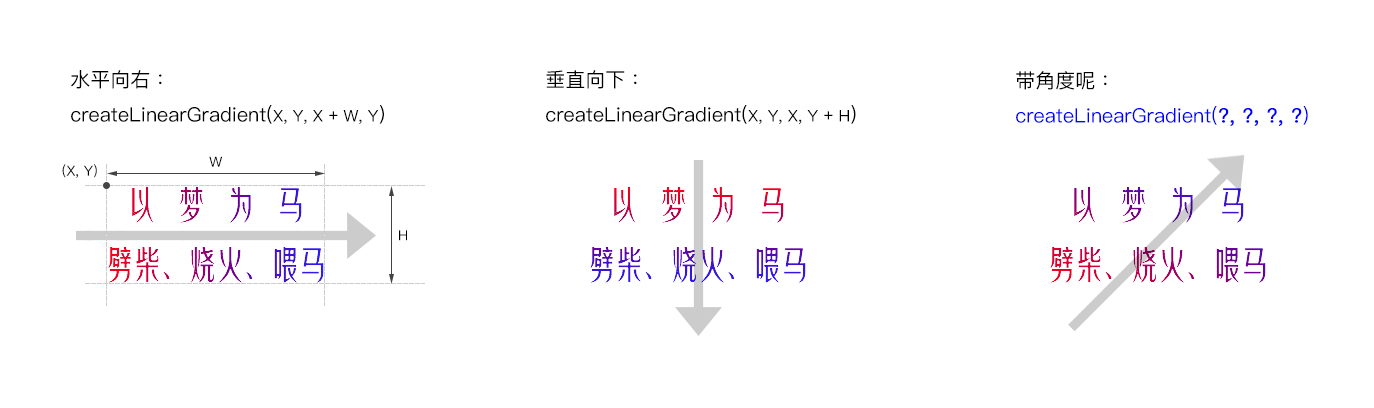### 猜想与答案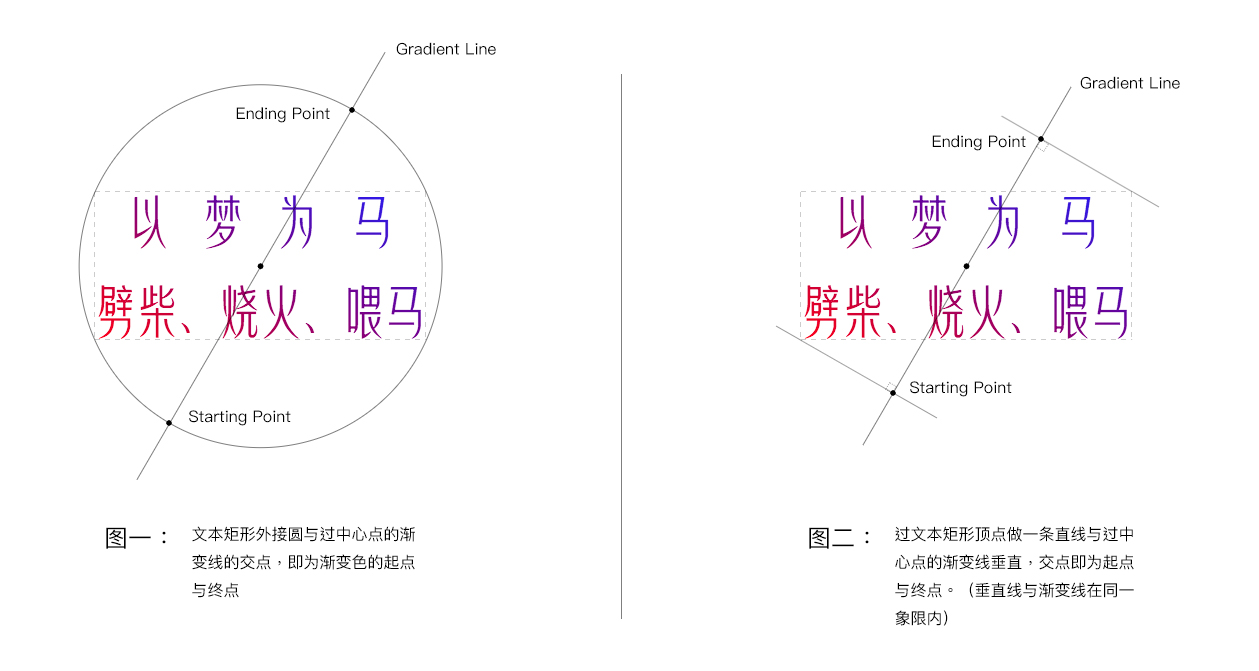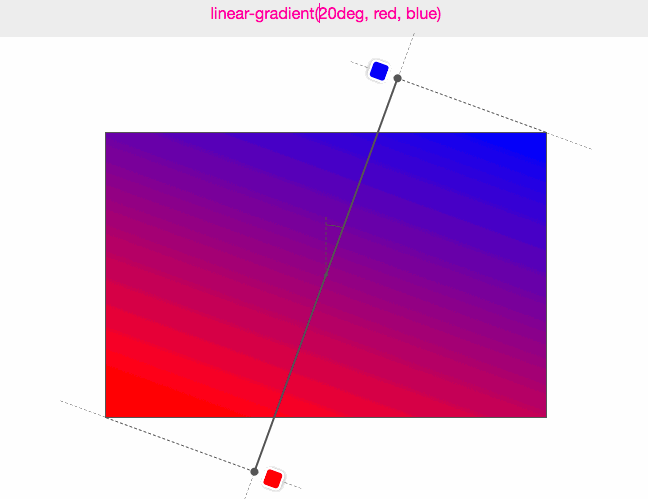(图来源：Do you really know CSS linear-gradients）

### 渐变起点与终点坐标的计算

1. 先求得起点与终点的长度（距离）。
2. 根据长度与文本矩形的中心点坐标分别计算出起点与终点坐标。

gradientLineLength = abs(W * sin(A)) + abs(H * cos(A)) 

gradientLineLength = abs(W * cos(A)) + abs(H * sin(A))

// 半长：
halfGradientLineLength = (abs(W * cos(A)) + abs(H * sin(A))) / 2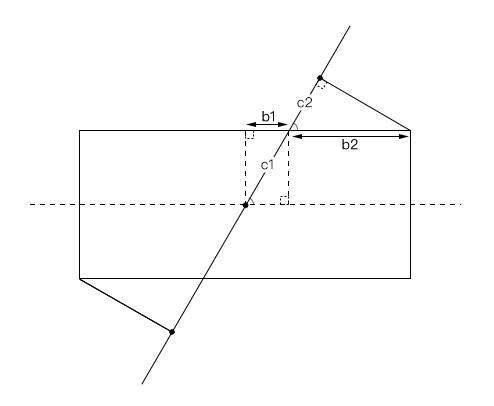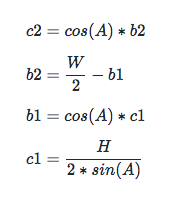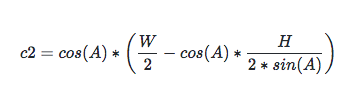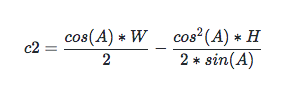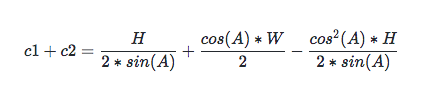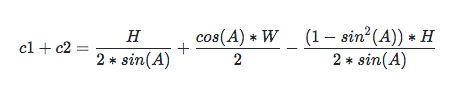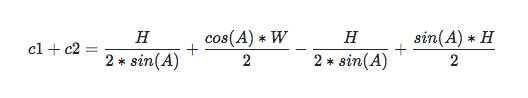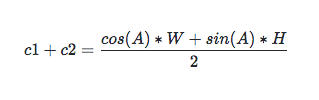centerX = X + W / 2
centerY = Y + H / 2 

startX = centerX - cos(A) * halfGradientLineLength
startY = centerY + sin(A) * halfGradientLineLength

endX = centerX + cos(A) * halfGradientLineLength
endY = centerY - sin(A) * halfGradientLineLength 

### 看看最终效果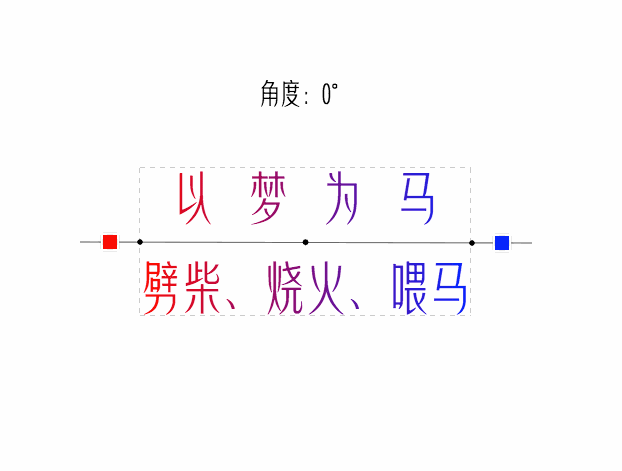### 经验注释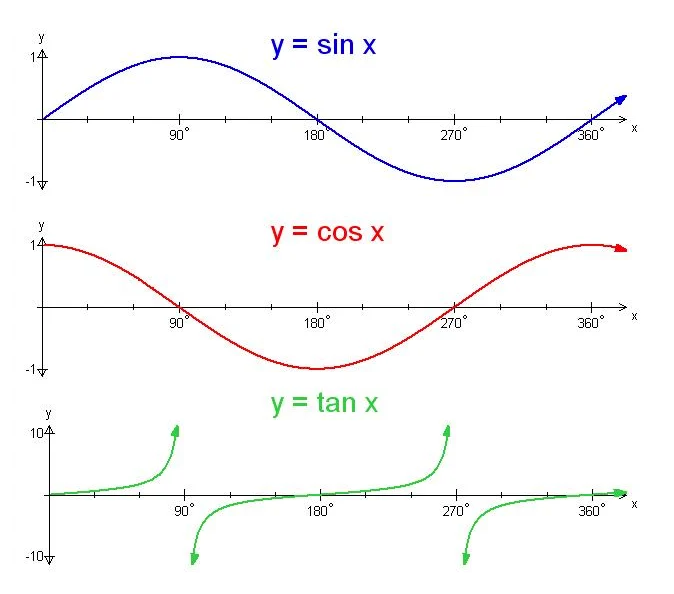### 参阅：

Do you really know CSS linear-gradients?